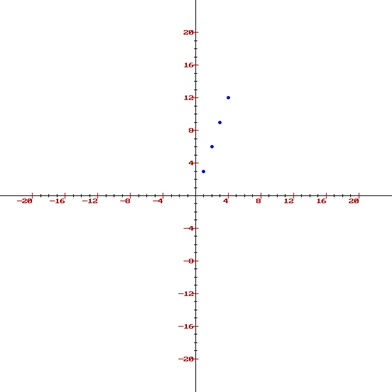# Direct Use of Line Equation in Computer Graphics

Computer Graphics | Direct Use of Line Equation: In this tutorial, we are going to learn about the Direct Use of Line Equation in Computer Graphics, 2D line and properties of good line drawing algorithms.
Submitted by Monika Sharma, on April 15, 2020

## Computer Graphics | Direct Use of Line Equation

The standard line equation, as we all know is used for drawing a line. It is given by: y = mx + c.

We are discussing here in 2D so we all know that there are 2 axes: x and y. Both of the axes are required to give the equation of any 2D shape. The line is a straight path joining 2 points in the x-y plane. If both the points are given then we can find the equation of a line.

### The slope of a line

The slope of a line defines the direction of a line. Its value is equal to the ratio of the difference of y coordinates and the difference. Assume that the two points are X( x1,y1 ) and Y( x2,y2 ). Its slope, 'm' will be: m = (y2 - y1) / (x2 - x1).

### Properties of Line Drawing Algorithm

The following are the properties that a line must hold in any line drawing algorithm,

1. Line must be straight
2. Line must terminate accurately
3. Line must have constant density
4. Density must be independent of its length
5. Line must be drawn very fast

### Line Drawing Algorithms

There are some set of rules and steps which help draw a line. These algorithms are given below,

1. Direct Use of line equation
2. DDA (Digital Differential Analyzer)
3. Bresenham's Algorithm

### Direct use of Line Equation

This is the simplest form of drawing a line. We all know that the equation of the line is y = mx + c. Here m is slope and c is the length from origin to the point where the line cuts y-axis. In this method, we will be having the start and endpoint of the line and by the help of that points, we'll calculate the other points which lie on the line. We have to find the slope of the line by using the given points.

We'll understand this better with the help of an example,

Example:

We have given two points X and Y. The coordinates of X are (0, 0) and the coordinates of Y are (5, 15). The slope of the line will be,

```    m = (15 - 0) / (5-0)
m = 3
```

We have the slope of the line. Now let us put the slope in the line equation.

```    y = 3x + c
```

Origin point is (0,0). So,

```    c = 0
```

Putting c=0 in the above equation.

```    y = 3x
```

Now we will calculate the intermediate points.

```    Let x = 1 ⟹ y = 3 x 1 ⟹ y = 3
Let x = 2 ⟹ y = 3 x 2 ⟹ y = 6
Let x = 3 ⟹ y = 3 x 3 ⟹ y = 9
Let x = 4 ⟹ y = 3 x 4 ⟹ y = 12
Let x = 5 ⟹ y = 3 x 5 ⟹ y = 15
```

We got the intermediate points which are, (1, 3), (2, 6), (3, 9), (4, 12) and finally (5, 15)

Now we'll plot these points on the graph.Hence, we have plotted the points that lie between the given points through the standard line equation. By doing so with a very small gap between these pints will give us the entire line.

Top MCQs

Languages: » C » C++ » C++ STL » Java » Data Structure » C#.Net » Android » Kotlin » SQL
Web Technologies: » PHP » Python » JavaScript » CSS » Ajax » Node.js » Web programming/HTML
Solved programs: » C » C++ » DS » Java » C#
Aptitude que. & ans.: » C » C++ » Java » DBMS
Interview que. & ans.: » C » Embedded C » Java » SEO » HR
CS Subjects: » CS Basics » O.S. » Networks » DBMS » Embedded Systems » Cloud Computing
» Machine learning » CS Organizations » Linux » DOS
More: » Articles » Puzzles » News/Updates